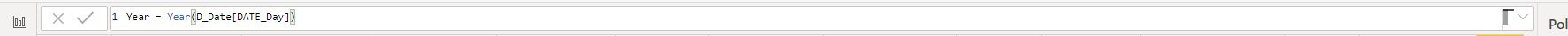• 11. 2. 2020
• Ing. Jan Zedníček - Data & Finance
• 0

DAX language (Data Analysis Expressions) servers for data management and editing prior reporting in Power BI or Powerpivot, similarly to function set in Excel. This language is able to return values based on use of functions. We can either enrich, add or analyze data prior reporting in Excel, and DAX enables us to do the same.

## Excel and DAX Functions Comparison – Friendly to Beginners

DAX language have an advantage for beginners since the visual similarity to Excel is significant – plenty of functions are named identically and do the same thing. But is I mentioned, there are also big differences between DAX and Excel. Let’s take a look at them.

### 1) What have Excel and DAX in Common?

• Purpose – both tools help to work with data and add or analyze them based on formulas
• Similar functions – many functions have identical names as in Excel (obviously in English) and functions can therefore be learned quicker. For example YEAR, WEEKDAY, AND, IF etc.
• Function categories – functions are, as in Excel, divided into categories which are pretty similar to each other. Below are listed categories and examples of the functions. You will see identical/similar ones if you know Excel functions by heart.
• Date and time – DATE, EOMONTH, TODAY()
• Information – ISERROR, ISNUMBER, ISTEXT
• Logical – IF, IFERROR, AND
• Math – ABS, POWER, ROUND
• Statistical – AVERAGE, MAX, COUNTBLANK
• Text – CONCACENATE, LEFT, SEARCH
• (!) Time intelligence – ENDOFMONTH, NEXTQUARTER, DATESBETWEEN
• (!) Filter– DISTINCT, FILTER, RELATEDTABLE

### 2) Differences of Excel and DAX

• DAX functions work as in a database apart from the Excel – we are used to percept excel cell or area as a calculation unit or a reference for a formula in Excel or other table processor. For example, we can have different formulas in one column. It is not possible like that in DAX. The formula always influences the whole column and each row of the table haves the same formula.
• DAX can return table as a result of the function
• Primary determination – DAX is used when working with table and columns whereas Excel works with values
• Data model – We need to set table references (column connections) if we work with multiple tables in powerpivot. This connection must be set up if we use DAX function which aggregates or searches for value in a different table.

## How and Where Should We Write Formulas in DAX?

First import some data (i.e. from excel) to Power BI desktop. We will get to the command line for formulas by opening imported table and creating new column. Example to demonstrate this is shown below. We work with the calendar table and type in a simple function which calculates Year from “Date” field. You can learn how to work with formula parameters in article Parameters – Introduction into DAX expressions.Now you only need to widen your knowledge of functions and train DAX language for Power BI.

Rate this post
Tags:### Ing. Jan Zedníček - Data & Finance

My name is Jan Zedníček and I work as a freelancer. I have been working as a freelancer for many companies for more than 10 years.
I used to work as a financial controller, analyst and manager at many different companies in field of banking and manufacturing. When I am not at work, I like playing volleyball, chess, doing a workout in the gym and I enjoy tasting of best quality rums.
I am trying to summarize all my knowledge on this website not to forget them and to put them forward to anyone.